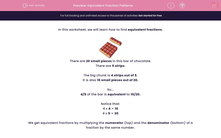# Equivalent Fraction Patterns

In this worksheet, students work with patterns when finding equivalent fractions.Key stage:  KS 2

Curriculum topic:   Maths and Numerical Reasoning

Curriculum subtopic:   Fractions

Popular topics:   Equivalent Fractions worksheets

Difficulty level:#### Worksheet Overview

In this worksheet, we will learn how to find equivalent fractions.There are 20 small pieces in this bar of chocolate.

There are 5 strips.

The big chunk is 4 strips out of 5.

It is also 16 small pieces out of 20.

So...

4/5 of the bar is equivalent to 16/20.

Notice that:

4 x 4 = 16

4 x 5 = 20

We get equivalent fractions by multiplying the numerator (top) and the denominator (bottom) of a fraction by the same number.

Example

Find the values of A, B, C and D to form equivalent fractions.

 3 9
=
 6 18
=
 A 27
=
 12 B
=
 C D

3/9 is the starting fraction.

In the second fraction, we get 6/18 by multiplying top and bottom of 3/9 by 2.

In the third fraction, we multiply top and bottom by 3. So A is 3 × 3 = 9.

In the fourth fraction, we multiply top and bottom by 4. So B is 4 × 9 = 36.

And in the last fraction, we multiply top and bottom by 5. So C is 5 × 3 = 15 and D is 5 × 9 = 45.

Notice the patterns.

The tops go  3, 6, 9, 12, 15.

The bottoms go 9, 18, 27, 36, 45.

In this worksheet, look for patterns like this.

### What is EdPlace?

We're your National Curriculum aligned online education content provider helping each child succeed in English, maths and science from year 1 to GCSE. With an EdPlace account you’ll be able to track and measure progress, helping each child achieve their best. We build confidence and attainment by personalising each child’s learning at a level that suits them.

Get started